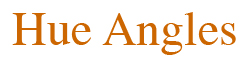## Monday, November 14, 2016

In the Spring 2016 issue (#474) of ISCC News, I described what appeared to be a paradox that emerged from an exercise for students : “If the wavelength of the green line of mercury is 546 nm in a vacuum, what is it in water? In heavy flint glass? [410 nm, 331 nm].” Would change of refractory material abutting the eye’s photoreceptors cause the color of a light to change as drastically as suggested here? More precisely, would changing the refractive index change the tristimulus values of a light with emitted spectral power distribution E(λ)?

Unlike in the wavelength domain, the change of refraction index would not affect the frequency of the transmitted light. I concluded that the proper choice of integration domain in which to perform tristimulus integration should be a matter of experiment.  This conclusion was incorrect. As often happens when one poses a paradox, closer examination provides a resolution. The tristimulus values should not change if the integrals are performed properly.  I describe the resolution in a tutorial note recently posted for publication in Color Research and Application .

It is reasonable and respectful of physical principles that a tristimulus value [say, M = the integral over λ of  E(λ) m(λ)] should not depend on the domain of integration used to obtain it. In  it is shown that the domain invariance from wavelength λ to frequency ν = c/λ is assured if the spectral power distribution of the test light in frequency is represented as E*(ν) =|dλ/dν| E(λ(ν)).

Now imagine a transformation from frequency to two alternative wavelength domains λ1 = c1/ν  and λ2 = c2/ν , where c1 and c2 represent speeds of light in different refractive indices (possibly functions of ν ).  Here, M doesn’t change either; rather, the spectral power distribution E*(ν) is multiplied by the Jacobian |dλ1/dν  |-1 or | dλ2/dν |-1, respectively. (Things get a bit more complicated when λ1 or λ2 is a nonmonotonic function of ν , but the principle is the same.) Of course, changing from λ1 to λ2 is equivalent to changing from λ1 to ν  and then from ν  to λ2.  There is no predicted variation of M, by construction.

So my “gentle paradox” evaporates by construction. Obeying the rules of mathematics leads back to where one started: tristimulus values don’t depend on the refractive index of the transmitted medium.

Where does that leave the role of experiment? If one were to measure the camera version of tristimulus values in different refractive media (say, with M being a measured camera value for a light E through a camera sensitivity function m), we would expect no change in those values.  But experiment can trump theory. If M were observed to change, then a new paradox would surface. From “paradox lost” we would see “paradox regained.”

References:
. HQ Fuller, RM. Fuller, and RG Fuller, Physics Including Human Applications. Harper and Row, 1978. Revised electronic version copyright 2009 by RG Fuller. http://physics.doane.edu/hpp/Resources/Fuller3/pdf/F3Chapter_19.pdf exercise 2, p. 436.
 MH Brill, Ways to define a tristimulus value. Color Res. Appl. 2016, Early View, DOI 10.1002/col.22079.

Michael H. Brill
Datacolor